### Learning Objectives

• Identify the form of an exponential function.
• Explain the difference between the graphs of $x^b$ and $b^x$.
• Recognize the significance of the number $e$.
• Identify the form of a logarithmic function.
• Explain the relationship between exponential and logarithmic functions.
• Describe how to calculate a logarithm to a different base.
• Identify the hyperbolic functions, their graphs, and basic identities.

In this section we examine exponential and logarithmic functions. We use the properties of these functions to solve equations involving exponential or logarithmic terms, and we study the meaning and importance of the number $e$. We also define hyperbolic and inverse hyperbolic functions, which involve combinations of exponential and logarithmic functions. (Note that we present alternative definitions of exponential and logarithmic functions in the chapter Applications of Integrations , and prove that the functions have the same properties with either definition.)

# Exponential Functions

Exponential functions arise in many applications. One common example is population growth .

For example, if a population starts with $P_0$ individuals and then grows at an annual rate of $2\%$, its population after 1 year is

$P(1)=P_0+0.02P_0=P_0(1+0.02)=P_0(1.02)$.

Its population after 2 years is

$P(2)=P(1)+0.02P(1)=P(1)(1.02)=P_0(1.02)^2$.

In general, its population after $t$ years is

$P(t)=P_0(1.02)^t$,

which is an exponential function. More generally, any function of the form $f(x)=b^x$, where $b < 0, \, b \ne 1$, is an exponential function with base $b$ and exponent $x$. Exponential functions have constant bases and variable exponents. Note that a function of the form $f(x)=x^b$ for some constant $b$ is not an exponential function but a power function.

To see the difference between an exponential function and a power function, we compare the functions $y=x^2$ and $y=2^x$. In (Figure) , we see that both $2^x$ and $x^2$ approach infinity as $x \to \infty$. Eventually, however, $2^x$ becomes larger than $x^2$ and grows more rapidly as $x \to \infty$. In the opposite direction, as $x \to -\infty, \, x^2 \to \infty$, whereas $2^x \to 0$. The line $y=0$ is a horizontal asymptote for $y=2^x$.

 $\mathbf{x}$ -3 -2 -1 0 1 2 3 4 5 6 $\mathbf{x^2}$ 9 4 1 0 1 4 9 16 25 36 $\mathbf{2^x}$ $1/8$ $1/4$ $1/2$ 1 2 4 8 16 32 64

In (Figure) , we graph both $y=x^2$ and $y=2^x$ to show how the graphs differ.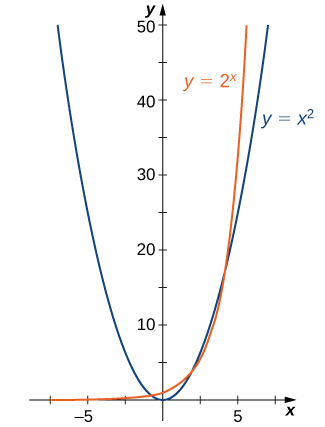Figure 1. Both $2^x$ and $x^2$ approach infinity as $x \to \infty$, but $2^x$ grows more rapidly than $x^2$. As $x \to -\infty, \, x^2 \to \infty$, whereas $2^x \to 0$.

## Evaluating Exponential Functions

Recall the properties of exponents: If $x$ is a positive integer, then we define $b^x=b\cdot b \cdots b$ (with $x$ factors of $b$). If $x$ is a negative integer, then $x=-y$ for some positive integer $y$, and we define $b^x=b^{-y}=1/b^y$. Also, $b^0$ is defined to be 1. If $x$ is a rational number, then $x=p/q$, where $p$ and $q$ are integers and $b^x=b^{p/q}=\sqrt[q]{b^p}$. For example, $9^{3/2}=\sqrt{9^3}=27$. However, how is $b^x$ defined if $x$ is an irrational number? For example, what do we mean by $2^{\sqrt{2}}$? This is too complex a question for us to answer fully right now; however, we can make an approximation. In (Figure) , we list some rational numbers approaching $\sqrt{2}$, and the values of $2^x$ for each rational number $x$ are presented as well. We claim that if we choose rational numbers $x$ getting closer and closer to $\sqrt{2}$, the values of $2^x$ get closer and closer to some number $L$. We define that number $L$ to be $2^{\sqrt{2}}$.

 $\mathbf{x}$ 1.4 1.41 1.414 1.4142 1.41421 1.41421 $\mathbf{2^x}$ 2.639 2.65737 2.66475 2.66512 2.66514 2.66514

### Bacterial Growth

Suppose a particular population of bacteria is known to double in size every 4 hours. If a culture starts with 1000 bacteria, the number of bacteria after 4 hours is $n(4)=1000\cdot 2$. The number of bacteria after 8 hours is $n(8)=n(4)\cdot 2=1000\cdot 2^2$. In general, the number of bacteria after $4m$ hours is $n(4m)=1000\cdot 2^m$. Letting $t=4m$, we see that the number of bacteria after $t$ hours is $n(t)=1000\cdot 2^{t/4}$. Find the number of bacteria after 6 hours, 10 hours, and 24 hours.

#### Solution

The number of bacteria after 6 hours is given by $n(6)=1000\cdot 2^{6/4} \approx 2828$ bacteria. The number of bacteria after 10 hours is given by $n(10)=1000\cdot 2^{10/4} \approx 5657$ bacteria. The number of bacteria after 24 hours is given by $n(24)=1000\cdot 2^6=64,000$ bacteria.

Given the exponential function $f(x)=100\cdot 3^{x/2}$, evaluate $f(4)$ and $f(10)$.

#### Solution

$f(4)=900; \, f(10)=24,300$.

Go to World Population Balance for another example of exponential population growth.

## Graphing Exponential Functions

For any base $b < 0, \, b\ne 1$, the exponential function $f(x)=b^x$ is defined for all real numbers $x$ and $b^x < 0$. Therefore, the domain of $f(x)=b^x$ is $(-\infty ,\infty)$ and the range is $(0,\infty)$. To graph $b^x$, we note that for $b < 1, \, b^x$ is increasing on $(-\infty ,\infty)$ and $b^x \to \infty$ as $x \to \infty$, whereas $b^x \to 0$ as $x \to -\infty$. On the other hand, if $0 < b < 1, \, f(x)=b^x$ is decreasing on $(-\infty ,\infty)$ and $b^x \to 0$ as $x \to \infty$ whereas $b^x \to \infty$ as $x \to -\infty$ ( (Figure) ).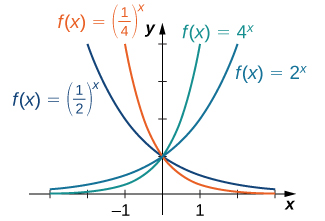Figure 2. If $b < 1$, then $b^x$ is increasing on $(-\infty ,\infty)$. If $0 Visit this site for more exploration of the graphs of exponential functions. Note that exponential functions satisfy the general laws of exponents. To remind you of these laws, we state them as rules. ### Rule: Laws of Exponents For any constants [latex]a > 0, \, b > 0$, and for all $x$ and $y$,

1. $b^x\cdot b^y=b^{x+y}$
2. $\large\frac{b^x}{b^y} \normalsize = b^{x-y}$
3. $(b^x)^y=b^{xy}$
4. $(ab)^x=a^x b^x$
5. $\large \frac{a^x}{b^x} \normalsize =(\frac{a}{b})^x$

### Using the Laws of Exponents

Use the laws of exponents to simplify each of the following expressions.

1. $\large \frac{(2x^{2/3})^3}{(4x^{-1/3})^2}$
2. $\large \frac{(x^3 y^{-1})^2}{(xy^2)^{-2}}$

#### Solution

1. We can simplify as follows:
$\large \frac{(2x^{2/3})^3}{(4x^{-1/3})^2} \normalsize = \large \frac{2^3(x^{2/3})^3}{4^2(x^{-1/3})^2} \normalsize = \large \frac{8x^2}{16x^{-2/3}} \normalsize = \large \frac{x^2x^{2/3}}{2} \normalsize = \large \frac{x^{8/3}}{2}$.
2. We can simplify as follows:
$\large \frac{(x^3y^{-1})^2}{(xy^2)^{-2}} \normalsize = \large \frac{(x^3)^2(y^{-1})^2}{x^{-2}(y^2)^{-2}} \normalsize = \large \frac{x^6y^{-2}}{x^{-2}y^{-4}} \normalsize = x^6x^2y^{-2}y^4 = x^8y^2$.

Use the laws of exponents to simplify $\frac{6x^{-3}y^2}{12x^{-4}y^5}$.

#### Hint

$\frac{x^a}{x^b}=x^{a-b}$

#### Solution

$\frac{x}{2y^3}$

# The Number  $e$

A special type of exponential function appears frequently in real-world applications. To describe it, consider the following example of exponential growth, which arises from compounding interest in a savings account. Suppose a person invests $P$ dollars in a savings account with an annual interest rate $r$, compounded annually. The amount of money after 1 year is

$A(1)=P+rP=P(1+r)$.

The amount of money after 2 years is

$A(2)=A(1)+rA(1)=P(1+r)+rP(1+r)=P(1+r)^2$.

More generally, the amount after $t$ years is

$A(t)=P(1+r)^t$.

If the money is compounded 2 times per year, the amount of money after half a year is

$A(\frac{1}{2})=P+(\frac{r}{2})P=P(1+(\frac{r}{2}))$.

The amount of money after 1 year is

$A(1)=A(\frac{1}{2})+(\frac{r}{2})A(\frac{1}{2})=P(1+\frac{r}{2})+\frac{r}{2}(P(1+\frac{r}{2}))=P(1+\frac{r}{2})^2$.

After $t$ years, the amount of money in the account is

$A(t)=P(1+\frac{r}{2})^{2t}$.

More generally, if the money is compounded $n$ times per year, the amount of money in the account after $t$ years is given by the function

$A(t)=P(1+\frac{r}{n})^{nt}$.

What happens as $n\to \infty$? To answer this question, we let $m=n/r$ and write

$(1+\frac{r}{n})^{nt}=(1+\frac{1}{m})^{mrt}$,

and examine the behavior of $(1+1/m)^m$ as $m\to \infty$, using a table of values ( (Figure) ).

 $\mathbf{m}$ 10 100 1000 10,000 100,000 1,000,000 $\mathbf{(1+\frac{1}{m})^m}$ 2.5937 2.7048 2.71692 2.71815 2.718268 2.718280

Looking at this table, it appears that $(1+1/m)^m$ is approaching a number between 2.7 and 2.8 as $m\to \infty$. In fact, $(1+1/m)^m$ does approach some number as $m\to \infty$. We call this number $e$. To six decimal places of accuracy,

$e \approx 2.718282$.

The letter $e$ was first used to represent this number by the Swiss mathematician Leonhard Euler during the 1720s. Although Euler did not discover the number, he showed many important connections between $e$ and logarithmic functions. We still use the notation $e$ today to honor Euler’s work because it appears in many areas of mathematics and because we can use it in many practical applications.

Returning to our savings account example, we can conclude that if a person puts $P$ dollars in an account at an annual interest rate $r$, compounded continuously, then $A(t)=Pe^{rt}$. This function may be familiar. Since functions involving base $e$ arise often in applications, we call the function $f(x)=e^x$ the natural exponential function . Not only is this function interesting because of the definition of the number $e$, but also, as discussed next, its graph has an important property.

Since $e < 1$, we know $e^x$ is increasing on $(-\infty ,\infty)$. In (Figure) , we show a graph of $f(x)=e^x$ along with a tangent line to the graph of at $x=0$. We give a precise definition of tangent line in the next chapter; but, informally, we say a tangent line to a graph of $f$ at $x=a$ is a line that passes through the point $(a,f(a))$ and has the same “slope” as $f$ at that point. The function $f(x)=e^x$ is the only exponential function $b^x$ with tangent line at $x=0$ that has a slope of 1. As we see later in the text, having this property makes the natural exponential function the simplest exponential function to use in many instances.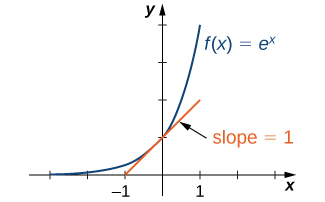Figure 3. The graph of $f(x)=e^x$ has a tangent line with slope 1 at $x=0$.

### Compounding Interest

Suppose $\500$ is invested in an account at an annual interest rate of $r=5.5\%$, compounded continuously.

1. Let $t$ denote the number of years after the initial investment and $A(t)$ denote the amount of money in the account at time $t$. Find a formula for $A(t)$.
2. Find the amount of money in the account after 10 years and after 20 years.

#### Solution

1. If $P$ dollars are invested in an account at an annual interest rate $r$, compounded continuously, then $A(t)=Pe^{rt}$. Here $P=\500$ and $r=0.055$. Therefore, $A(t)=500e^{0.055t}$.
2. After 10 years, the amount of money in the account is
$A(10)=500e^{0.055\cdot 10}=500e^{0.55}\approx \866.63$.

After 20 years, the amount of money in the account is

$A(20)=500e^{0.055\cdot 20}=500e^{1.1}\approx \1,502.08$.

If $\750$ is invested in an account at an annual interest rate of $4\%$, compounded continuously, find a formula for the amount of money in the account after $t$ years. Find the amount of money after 30 years.

#### Hint

$A(t)=Pe^{rt}$.

Solution:

$A(t)=750e^{0.04t}$. After 30 years, there will be approximately $\2,490.09$.

# Logarithmic Functions

Using our understanding of exponential functions, we can discuss their inverses, which are the logarithmic functions. These come in handy when we need to consider any phenomenon that varies over a wide range of values, such as pH in chemistry or decibels in sound levels.

The exponential function $f(x)=b^x$ is one-to-one, with domain $(-\infty ,\infty)$ and range $(0,\infty )$. Therefore, it has an inverse function, called the logarithmic function with base $b$. For any $b < 0, \, b \ne 1$, the logarithmic function with base $b$, denoted $\log_b$, has domain $(0,\infty )$ and range $(-\infty ,\infty )$, and satisfies

$\log_b(x)=y$ if and only if $b^y=x$.

For example,

$\begin{array}{cccc} \log_2 (8)=3\hfill & & & \text{since}\phantom{\rule{3em}{0ex}}2^3=8,\hfill \\ \log_{10} (\frac{1}{100})=-2\hfill & & & \text{since}\phantom{\rule{3em}{0ex}}10^{-2}=\frac{1}{10^2}=\frac{1}{100},\hfill \\ \log_b (1)=0\hfill & & & \text{since}\phantom{\rule{3em}{0ex}}b^0=1 \, \text{for any base} \, b < 0.\hfill \end{array}$

Furthermore, since $y=\log_b (x)$ and $y=b^x$ are inverse functions,

$\log_b (b^x)=x \, \text{and} \, b^{\log_b (x)}=x$.

The most commonly used logarithmic function is the function $\log_e (x)$. Since this function uses natural $e$ as its base, it is called the natural logarithm . Here we use the notation $\ln(x)$ or $\ln x$ to mean $\log_e (x)$. For example,

$\ln (e)=\log_e (e)=1, \, \ln(e^3)=\log_e (e^3)=3, \, \ln(1)=\log_e (1)=0$.

Since the functions $f(x)=e^x$ and $g(x)=\ln(x)$ are inverses of each other,

$\ln(e^x)=x \, \text{and} \, e^{\ln x}=x$,

and their graphs are symmetric about the line $y=x$ ( (Figure) ).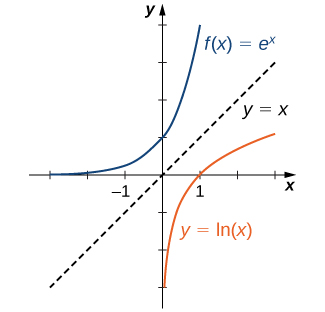Figure 4: The functions $y=e^x$ and $y=\ln(x)$ are inverses of each other, so their graphs are symmetric about the line $y=x$.

At this site you can see an example of a base-10 logarithmic scale.

In general, for any base $b < 0, \, b\ne 1$, the function $g(x)=\log_b (x)$ is symmetric about the line $y=x$ with the function $f(x)=b^x$. Using this fact and the graphs of the exponential functions, we graph functions $\log_b (x)$ for several values of $b < 1$ ( (Figure) ).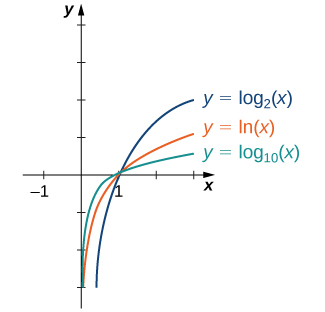Figure 5: Graphs of $y=\log_b (x)$ are depicted for $b=2, \, e, \, 10$.

Before solving some equations involving exponential and logarithmic functions, let’s review the basic properties of logarithms.

### Rule: Properties of Logarithms

If $a,b,c < 0, \, b\ne 1$, and $r$ is any real number, then

$\begin{array}{cccc}1.\phantom{\rule{2em}{0ex}}\log_b (ac)=\log_b (a)+\log_b (c)\hfill & & & \text{(Product property)}\hfill \\ 2.\phantom{\rule{2em}{0ex}}\log_b(\frac{a}{c})=\log_b (a) -\log_b (c)\hfill & & & \text{(Quotient property)}\hfill \\ 3.\phantom{\rule{2em}{0ex}}\log_b (a^r)=r \log_b (a)\hfill & & & \text{(Power property)}\hfill \end{array}$

### Solving Equations Involving Exponential Functions

Solve each of the following equations for $x$.

1. $5^x=2$
2. $e^x+6e^{-x}=5$

#### Solution

1. Applying the natural logarithm function to both sides of the equation, we have
$\ln 5^x=\ln 2$.

Using the power property of logarithms,

$x \ln 5=\ln 2$.

Therefore, $x=\ln 2 / \ln 5$.

2. Multiplying both sides of the equation by $e^x$, we arrive at the equation
$e^{2x}+6=5e^x$.

Rewriting this equation as

$e^{2x}-5e^x+6=0$,

we can then rewrite it as a quadratic equation in $e^x$:

$(e^x)^2-5(e^x)+6=0$.

Now we can solve the quadratic equation. Factoring this equation, we obtain

$(e^x-3)(e^x-2)=0$.

Therefore, the solutions satisfy $e^x=3$ and $e^x=2$. Taking the natural logarithm of both sides gives us the solutions $x=\ln 3, \, \ln 2$.

Solve $e^{2x} / (3+e^{2x})=1/2$.

#### Hint

First solve the equation for $e^{2x}$.

#### Solution

$x=\frac{\ln 3}{2}$

### Solving Equations Involving Logarithmic Functions

Solve each of the following equations for $x$.

1. $\ln (\frac{1}{x})=4$
2. $\log_{10} \sqrt{x}+ \log_{10} x=2$
3. $\ln(2x)-3 \ln(x^2)=0$

#### Solution

1. By the definition of the natural logarithm function,
$\ln\big(\frac{1}{x}\big)=4 \, \text{if and only if} \, e^4=\frac{1}{x}$.

Therefore, the solution is $x=1/e^4$.

2. Using the product and power properties of logarithmic functions, rewrite the left-hand side of the equation as
$\log_{10} \sqrt{x}+ \log_{10} x = \log_{10} x \sqrt{x} = \log_{10}x^{3/2} = \frac{3}{2} \log_{10} x$.

Therefore, the equation can be rewritten as

$\frac{3}{2} \log_{10} x = 2 \, \text{or} \, \log_{10} x = \frac{4}{3}$.

The solution is $x=10^{4/3}=10\sqrt{10}$.

3. Using the power property of logarithmic functions, we can rewrite the equation as $\ln(2x) - \ln(x^6) = 0$.
Using the quotient property, this becomes

$\ln\big(\frac{2}{x^5}\big)=0$.

Therefore, $2/x^5=1$, which implies $x=\sqrt{2}$. We should then check for any extraneous solutions.

Solve $\ln(x^3)-4 \ln (x)=1$.

#### Hint

First use the power property, then use the product property of logarithms.

#### Solution

$x=\frac{1}{e}$

When evaluating a logarithmic function with a calculator, you may have noticed that the only options are $\log_{10}$ or log, called the common logarithm , or ln, which is the natural logarithm. However, exponential functions and logarithm functions can be expressed in terms of any desired base $b$. If you need to use a calculator to evaluate an expression with a different base, you can apply the change-of-base formulas first. Using this change of base, we typically write a given exponential or logarithmic function in terms of the natural exponential and natural logarithmic functions.

### Rule: Change-of-Base Formulas

Let $a < 0, \, b < 0$, and $a\ne 1, \, b\ne 1$.

1. $a^x=b^{x \log_b a}$ for any real number $x$.
If $b=e$, this equation reduces to $a^x=e^{x \log_e a}=e^{x \ln a}$.
2. $\log_a x=\frac{\log_b x}{\log_b a}$ for any real number $x < 0$. If $b=e$, this equation reduces to $\log_a x=\frac{\ln x}{\ln a}$.

## Proof

For the first change-of-base formula, we begin by making use of the power property of logarithmic functions. We know that for any base $b < 0, \, b\ne 1, \, \log_b (a^x)=x \log_b a$. Therefore,

$b^{\log_b(a^x)}=b^{x \log_b a}$.

In addition, we know that $b^x$ and $\log_b (x)$ are inverse functions. Therefore,

$b^{\log_b (a^x)}=a^x$.

Combining these last two equalities, we conclude that $a^x=b^{x \log_b a}$.

To prove the second property, we show that

$(\log_b a)\cdot (\log_a x)=\log_b x$.

Let $u=\log_b a, \, v=\log_a x$, and $w=\log_b x$. We will show that $u\cdot v=w$. By the definition of logarithmic functions, we know that $b^u=a, \, a^v=x$, and $b^w=x$. From the previous equations, we see that

$b^{uv}=(b^u)^v=a^v=x=b^w$.

Therefore, $b^{uv}=b^w$. Since exponential functions are one-to-one, we can conclude that $u\cdot v=w$.

### Changing Bases

Use a calculating utility to evaluate $\log_3 7$ with the change-of-base formula presented earlier.

#### Solution

Use the second equation with $a=3$ and $e=3$:

$\log_3 7=\frac{\ln 7}{\ln 3} \approx 1.77124$.

Use the change-of-base formula and a calculating utility to evaluate $\log_4 6$.

#### Hint

Use the change of base to rewrite this expression in terms of expressions involving the natural logarithm function.

1.29248

### Chapter Opener: The Richter Scale for Earthquakes

In 1935, Charles Richter developed a scale (now known as the Richter scale ) to measure the magnitude of an earthquake . The scale is a base-10 logarithmic scale, and it can be described as follows: Consider one earthquake with magnitude $R_1$ on the Richter scale and a second earthquake with magnitude $R_2$ on the Richter scale. Suppose $R_1 < R_2$, which means the earthquake of magnitude $R_1$ is stronger, but how much stronger is it than the other earthquake? A way of measuring the intensity of an earthquake is by using a seismograph to measure the amplitude of the earthquake waves. If $A_1$ is the amplitude measured for the first earthquake and $A_2$ is the amplitude measured for the second earthquake, then the amplitudes and magnitudes of the two earthquakes satisfy the following equation:

$R_1 - R_2 = \log_{10}(\frac{A_1}{A_2})$.

Consider an earthquake that measures 8 on the Richter scale and an earthquake that measures 7 on the Richter scale. Then,

$8-7=\log_{10}(\frac{A_1}{A_2})$.

Therefore,

$\log_{10}(\frac{A_1}{A_2})=1$,

which implies $A_1 / A_2 = 10$ or $A_1 = 10A_2$. Since $A_1$ is 10 times the size of $A_2$, we say that the first earthquake is 10 times as intense as the second earthquake. On the other hand, if one earthquake measures 8 on the Richter scale and another measures 6, then the relative intensity of the two earthquakes satisfies the equation

$\log_{10}(\frac{A_1}{A_2})=8-6=2$.

Therefore, $A_1=100A_2$. That is, the first earthquake is 100 times more intense than the second earthquake.

How can we use logarithmic functions to compare the relative severity of the magnitude 9 earthquake in Japan in 2011 with the magnitude 7.3 earthquake in Haiti in 2010?

#### Solution

To compare the Japan and Haiti earthquakes, we can use an equation presented earlier:

$9-7.3=\log_{10}(\frac{A_1}{A_2})$.

Therefore, $A_1 / A_2=10^{1.7}$, and we conclude that the earthquake in Japan was approximately 50 times more intense than the earthquake in Haiti.

Compare the relative severity of a magnitude 8.4 earthquake with a magnitude 7.4 earthquake.

#### Hint

$R_1-R_2=\log_{10}(A_1 / A_2)$.

Solution:

The magnitude 8.4 earthquake is roughly 10 times as severe as the magnitude 7.4 earthquake.

# Hyperbolic Functions

The hyperbolic functions are defined in terms of certain combinations of $e^x$ and $e^{-x}$. These functions arise naturally in various engineering and physics applications, including the study of water waves and vibrations of elastic membranes. Another common use for a hyperbolic function is the representation of a hanging chain or cable, also known as a catenary ( (Figure) ). If we introduce a coordinate system so that the low point of the chain lies along the $y$-axis, we can describe the height of the chain in terms of a hyperbolic function. First, we define the hyperbolic functions.Figure 7. The shape of a strand of silk in a spider’s web can be described in terms of a hyperbolic function. The same shape applies to a chain or cable hanging from two supports with only its own weight. (credit: “Mtpaley”, Wikimedia Commons)

### Definition

Hyperbolic cosine

$\cosh x=\large \frac{e^x+e^{-x}}{2}$

Hyperbolic sine

$\sinh x=\large \frac{e^x-e^{-x}}{2}$

Hyperbolic tangent

$\tanh x=\large \frac{\sinh x}{\cosh x} \normalsize = \large \frac{e^x-e^{-x}}{e^x+e^{-x}}$

Hyperbolic cosecant

$\text{csch} \, x=\large \frac{1}{\sinh x} \normalsize = \large \frac{2}{e^x-e^{-x}}$

Hyperbolic secant

$\text{sech} \, x=\large \frac{1}{\cosh x} \normalsize = \large \frac{2}{e^x+e^{-x}}$

Hyperbolic cotangent

$\coth x=\large \frac{\cosh x}{\sinh x} \normalsize = \large \frac{e^x+e^{-x}}{e^x-e^{-x}}$

The name cosh rhymes with “gosh,” whereas the name sinh is pronounced “cinch.” Tanh , sech , csch , and coth are pronounced “tanch,” “seech,” “coseech,” and “cotanch,” respectively.

Using the definition of $\cosh(x)$ and principles of physics, it can be shown that the height of a hanging chain, such as the one in (Figure) , can be described by the function $h(x)=a \cosh(x/a)+c$ for certain constants $a$ and $c$.

But why are these functions called hyperbolic functions ? To answer this question, consider the quantity $\cosh^2 t-\sinh^2 t$. Using the definition of $\cosh$ and $\sinh$, we see that

$\cosh^2 t-\sinh^2 t=\large \frac{e^{2t}+2+e^{-2t}}{4}-\frac{e^{2t}-2+e^{-2t}}{4} \normalsize =1$.

This identity is the analog of the trigonometric identity $\cos^2 t+\sin^2 t=1$. Here, given a value $t$, the point $(x,y)=(\cosh t,\sinh t)$ lies on the unit hyperbola $x^2-y^2=1$ ( (Figure) ).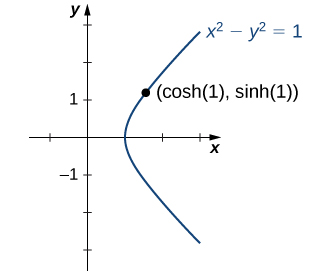Figure 8.The unit hyperbola $\cosh^2 t-\sinh^2 t=1$.

## Graphs of Hyperbolic Functions

To graph $\cosh x$ and $\sinh x$, we make use of the fact that both functions approach $(1/2)e^x$ as $x \to \infty$, since $e^{-x} \to 0$ as $x \to \infty$. As $x \to -\infty, \, \cosh x$ approaches $1/2e^{-x}$, whereas $\sinh x$ approaches $-1/2e^{-x}$. Therefore, using the graphs of $1/2e^x, \, 1/2e^{-x}$, and $-1/2e^{-x}$ as guides, we graph $\cosh x$ and $\sinh x$. To graph $\tanh x$, we use the fact that $\tanh(0)=1, \, -1<\tanh(x)<1$ for all $x, \, \tanh x \to 1$ as $x \to \infty$, and $\tanh x \to -1$ as $x \to -\infty$. The graphs of the other three hyperbolic functions can be sketched using the graphs of $\cosh x, \, \sinh x$, and $\tanh x$ ( (Figure) ).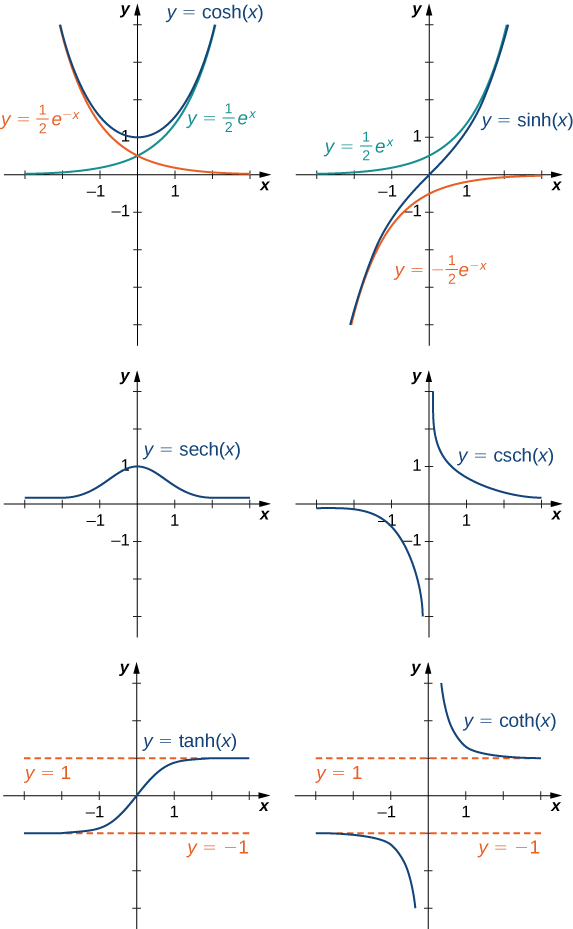Figure 9. The hyperbolic functions involve combinations of $e^x$ and $e^{-x}$.

## Identities Involving Hyperbolic Functions

The identity $\cosh^2 t-\sinh^2 t$, shown in (Figure) , is one of several identities involving the hyperbolic functions, some of which are listed next. The first four properties follow easily from the definitions of hyperbolic sine and hyperbolic cosine. Except for some differences in signs, most of these properties are analogous to identities for trigonometric functions.

### Rule: Identities Involving Hyperbolic Functions

1. $\cosh(-x)=\cosh x$
2. $\sinh(-x)=-\sinh x$
3. $\cosh x+\sinh x=e^x$
4. $\cosh x-\sinh x=e^{-x}$
5. $\cosh^2 x-\sinh^2 x=1$
6. $1-\tanh^2 x=\text{sech}^2 x$
7. $\coth^2 x-1=\text{csch}^2 x$
8. $\sinh(x \pm y)=\sinh x \cosh y \pm \cosh x \sinh y$
9. $\cosh (x \pm y)=\cosh x \cosh y \pm \sinh x \sinh y$

### Evaluating Hyperbolic Functions

1. Simplify $\sinh(5 \ln x)$.
2. If $\sinh x=3/4$, find the values of the remaining five hyperbolic functions.

#### Solution

1. Using the definition of the $\sinh$ function, we write
$\sinh(5 \ln x)=\large \frac{e^{5 \ln x}-e^{-5 \ln x}}{2} \normalsize = \large \frac{e^{\ln(x^5)}-e^{\ln(x^{-5})}}{2} \normalsize =\large \frac{x^5-x^{-5}}{2}$.
2. Using the identity $\cosh^2 x-\sinh^2 x=1$, we see that
$\cosh^2 x=1+\big(\frac{3}{4}\big)^2=\frac{25}{16}$.

Since $\cosh x \ge 1$ for all $x$, we must have $\cosh x=5/4$. Then, using the definitions for the other hyperbolic functions, we conclude that $\tanh x=3/5, \, \text{csch} \, x=4/3, \, \text{sech} \, x=4/5$, and $\coth x=5/3$.

Simplify $\cosh(2 \ln x)$.

#### Hint

Use the definition of the cosh function and the power property of logarithm functions.

#### Solution

$(x^2+x^{-2})/2$

## Inverse Hyperbolic Functions

From the graphs of the hyperbolic functions, we see that all of them are one-to-one except $\cosh x$ and $\text{sech} \, x$. If we restrict the domains of these two functions to the interval $[0,\infty)$, then all the hyperbolic functions are one-to-one, and we can define the inverse hyperbolic functions. Since the hyperbolic functions themselves involve exponential functions, the inverse hyperbolic functions involve logarithmic functions.

### Definition

Inverse Hyperbolic Functions

$\begin{array}{cccc}\sinh^{-1} x=\text{arcsinh } x=\ln(x+\sqrt{x^2+1})\hfill & & & \cosh^{-1} x=\text{arccosh } x=\ln(x+\sqrt{x^2-1})\hfill \\ \tanh^{-1} x=\text{arctanh } x=\frac{1}{2}\ln\big(\frac{1+x}{1-x}\big)\hfill & & & \coth^{-1} x=\text{arccot } x=\frac{1}{2}\ln\big(\frac{x+1}{x-1}\big)\hfill \\ \text{sech}^{-1} x=\text{arcsech } x=\ln\big(\frac{1+\sqrt{1-x^2}}{x}\big)\hfill & & & \text{csch}^{-1} x=\text{arccsch } x=\ln\big(\frac{1}{x}+\frac{\sqrt{1+x^2}}{|x|}\big)\hfill \end{array}$

Let’s look at how to derive the first equation. The others follow similarly. Suppose $y=\sinh^{-1} x$. Then, $x=\sinh y$ and, by the definition of the hyperbolic sine function, $x=\frac{e^y-e^{-y}}{2}$. Therefore,

$e^y-2x-e^{-y}=0$.

Multiplying this equation by $e^y$, we obtain

$e^{2y}-2xe^y-1=0$.

This can be solved like a quadratic equation, with the solution

$e^y=\large \frac{2x \pm \sqrt{4x^2+4}}{2} \normalsize =x \pm \sqrt{x^2+1}$.

Since $e^y < 0$, the only solution is the one with the positive sign. Applying the natural logarithm to both sides of the equation, we conclude that

$y=\ln(x+\sqrt{x^2+1})$.

### Evaluating Inverse Hyperbolic Functions

Evaluate each of the following expressions.

$\sinh^{-1}(2)$
$\tanh^{-1}(1/4)$

#### Solution

$\sinh^{-1}(2)=\ln(2+\sqrt{2^2+1})=\ln(2+\sqrt{5}) \approx 1.4436$

$\tanh^{-1}(1/4)=\frac{1}{2}\ln(\frac{1+1/4}{1-1/4})=\frac{1}{2}\ln(\frac{5/4}{3/4})=\frac{1}{2}\ln(\frac{5}{3}) \approx 0.2554$

Evaluate $\tanh^{-1}(1/2)$.

#### Hint

Use the definition of $\tanh^{-1} x$ and simplify.

#### Solution

$\frac{1}{2}\ln(3) \approx 0.5493$.

### Key Concepts

• The exponential function $y=b^x$ is increasing if $b < 1$ and decreasing if $0 < b < 1$. Its domain is $(-\infty ,\infty)$ and its range is $(0,\infty)$.
• The logarithmic function $y=\log_b(x)$ is the inverse of $y=b^x$. Its domain is $(0,\infty)$ and its range is $(-\infty,\infty)$.
• The natural exponential function is $y=e^x$ and the natural logarithmic function is $y=\ln x=\log_e x$.
• Given an exponential function or logarithmic function in base $a$, we can make a change of base to convert this function to any base $b < 0, \, b \ne 1$. We typically convert to base $e$.
• The hyperbolic functions involve combinations of the exponential functions $e^x$ and $e^{-x}$. As a result, the inverse hyperbolic functions involve the natural logarithm.

For the following exercises, evaluate the given exponential functions as indicated, accurate to two significant digits after the decimal.

1.  $f(x)=5^x$

a. $x=3$ b. $x=\frac{1}{2}$ c. $x=\sqrt{2}$

#### Solution

a. 125 b. 2.24 c. 9.74

2.  $f(x)=(0.3)^x$

a. $x=-1$ b. $x=4$ c. $x=-1.5$

3.  $f(x)=10^x$

a. $x=-2$ b. $x=4$ c. $x=\frac{5}{3}$

#### Solution

a. 0.01 b. 10,000 c. 46.42

4.  $f(x)=e^x$

a. $x=2$ b. $x=-3.2$ c. $x=\pi$

For the following exercises, match the exponential equation to the correct graph.

1. $y=4^{-x}$
2. $y=3^{x-1}$
3. $y=2^{x+1}$
4. $y=(\frac{1}{2})^x+2$
5. $y=-3^{-x}$
6. $y=1-5^x$
5.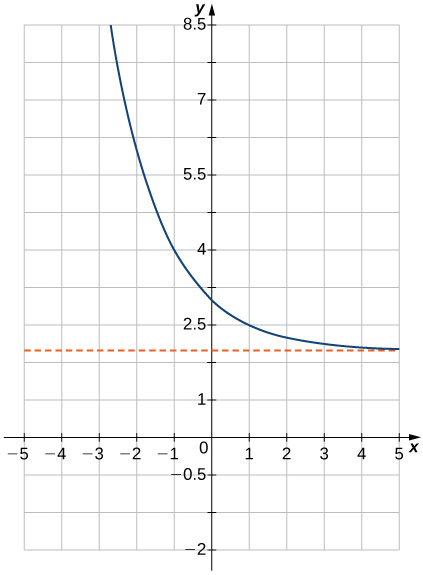#### Solution

d

6.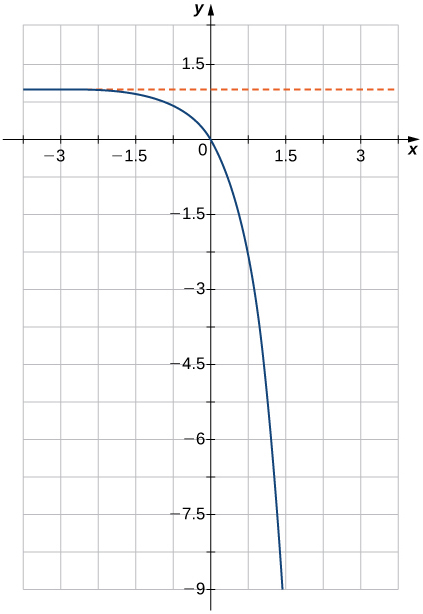7.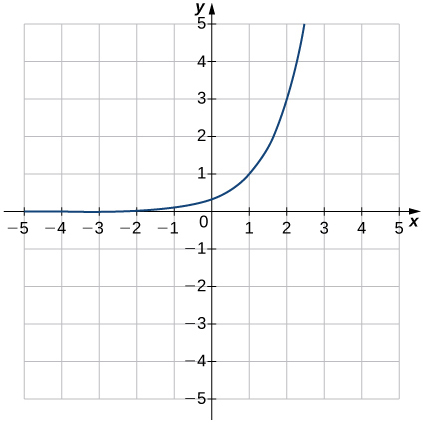#### Solution

b

8.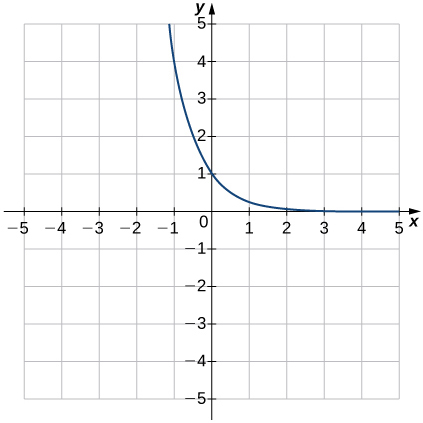9.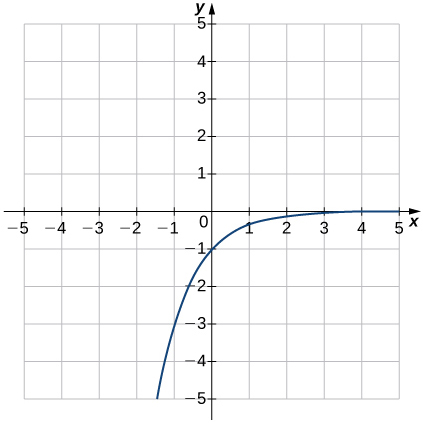#### Solution

e

10.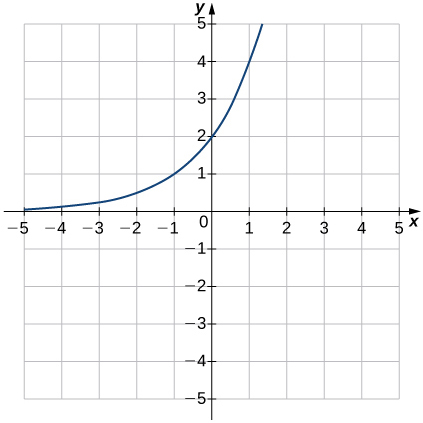For the following exercises, sketch the graph of the exponential function. Determine the domain, range, and horizontal asymptote.

11.  $f(x)=e^x+2$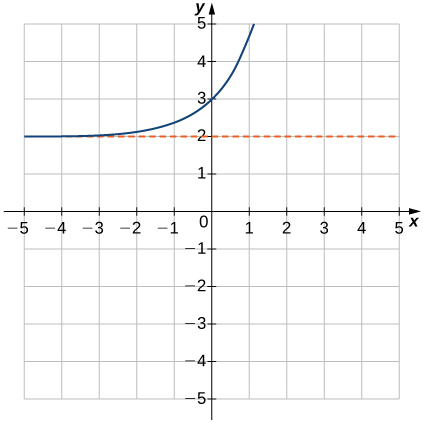#### Solution

Domain: all real numbers, Range: $(2,\infty)$, Horizontal asymptote at $y=2$

12.  $f(x)=-2^x$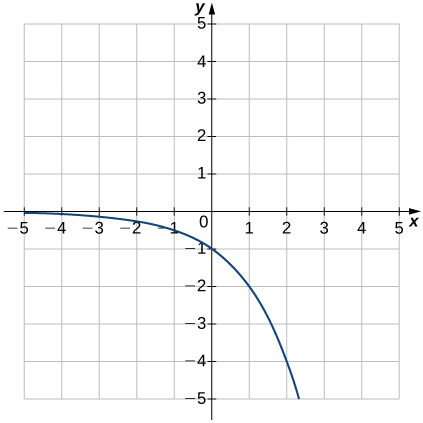13.  $f(x)=3^{x+1}$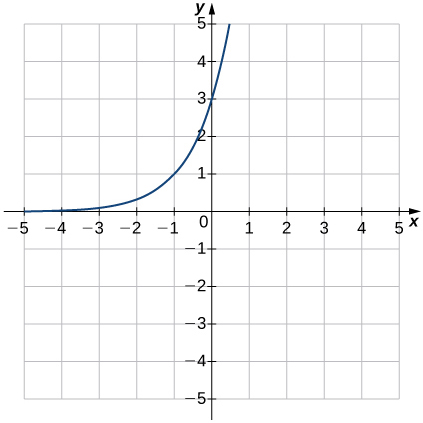#### Solution

Domain: all real numbers, Range: $(0,\infty)$, Horizontal asymptote at $y=0$

14.  $f(x)=4^x-1$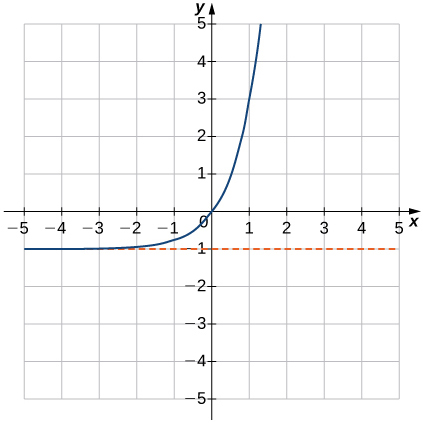15.  $f(x)=1-2^{-x}$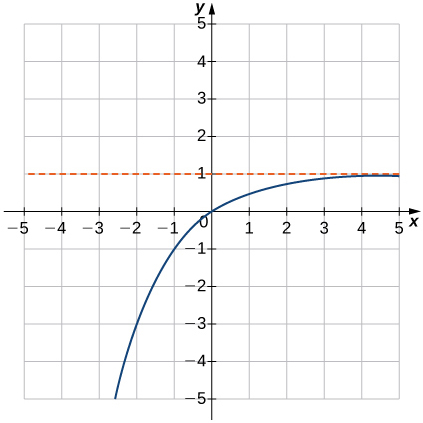#### Solution

Domain: all real numbers, Range: $(-\infty ,1)$, Horizontal asymptote at $y=1$

16.  $f(x)=5^{x+1}+2$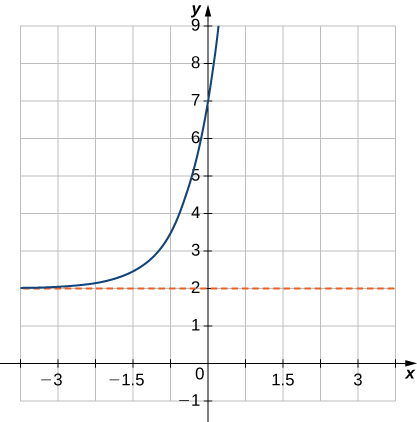17.  $f(x)=e^{-x}-1$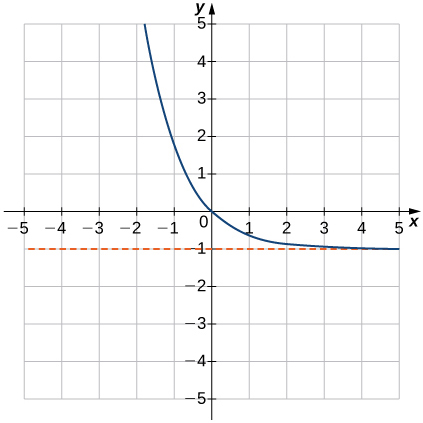#### Solution

Domain: all real numbers, Range: $(-1,\infty )$, Horizontal asymptote at $y=-1$

For the following exercises, write the equation in equivalent exponential form.

18.  $\log_3 81=4$

19.  $\log_8 2=\frac{1}{3}$

#### Solution

$8^{1/3}=2$

20.  $\log_5 1=0$

21.  $\log_5 25=2$

#### Solution

$5^2=25$

22.  $\log 0.1=-1$

23.  $\ln(\frac{1}{e^3})=-3$

#### Solution

$e^{-3}=\frac{1}{e^3}$

24.  $\log_9 3=0.5$

25.  $\ln 1=0$

#### Solution

$e^0=1$

For the following exercises, write the equation in equivalent logarithmic form.

26.  $2^3=8$

27.  $4^{-2}=\frac{1}{16}$

#### Solution

$\log_4(\frac{1}{16})=-2$

28.  $10^2=100$

29.  $9^0=1$

#### Solution

$\log_9 1=0$

30.  $(\frac{1}{3})^3=\frac{1}{27}$

31.  $\sqrt{64}=4$

#### Solution

$\log_{64} 4=\frac{1}{3}$

32.  $e^x=y$

33.  $9^y=150$

#### Solution

$\log_9 150=y$

34.  $b^3=45$

35.  $4^{-3/2}=0.125$

#### Solution

$\log_4 0.125=-\frac{3}{2}$

For the following exercises, use the given graphs of the logarithmic functions to determine their domain, range, and vertical asymptote.

36.  $f(x)=3+\ln x$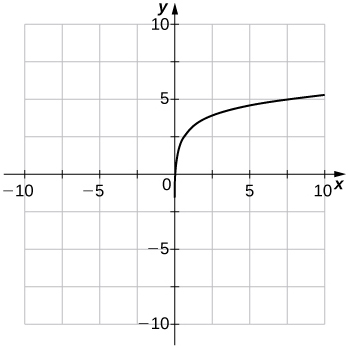37.  $f(x)=\ln(x-1)$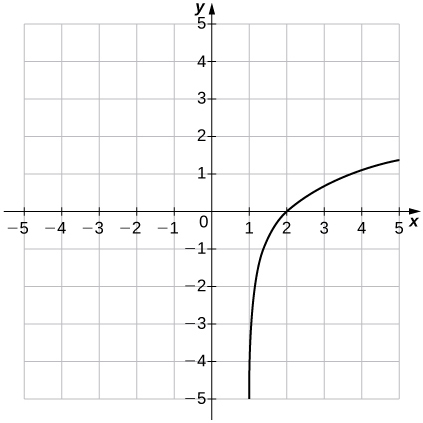#### Solution

Domain: $(1,\infty ),$ Range: $(-\infty ,\infty)$, Vertical asymptote at $x=1$

38.  $f(x)=\ln(-x)$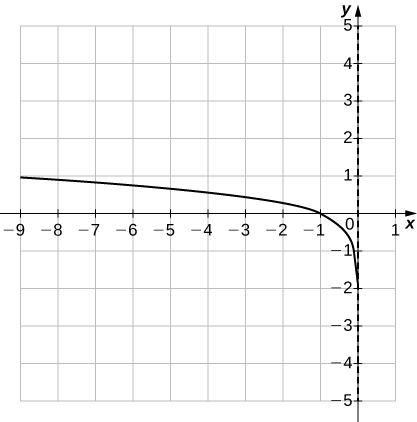39.  $f(x)=1-\ln x$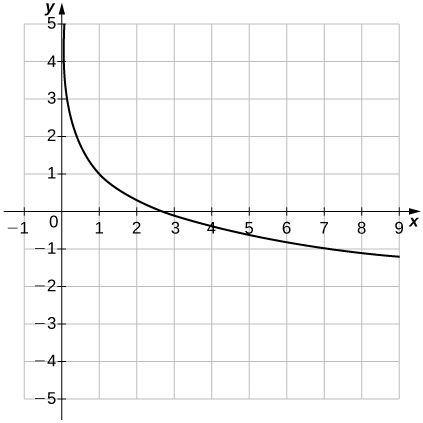#### Solution

Domain: $(0,\infty)$, Range: $(-\infty ,\infty)$, Vertical asymptote at $x=0$

40.  $f(x)=\log x-1$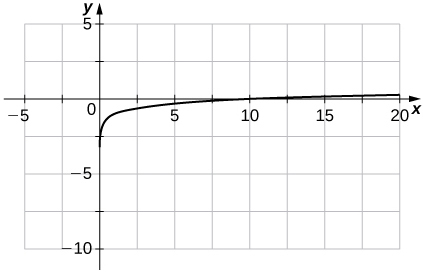41.  $f(x)=\ln(x+1)$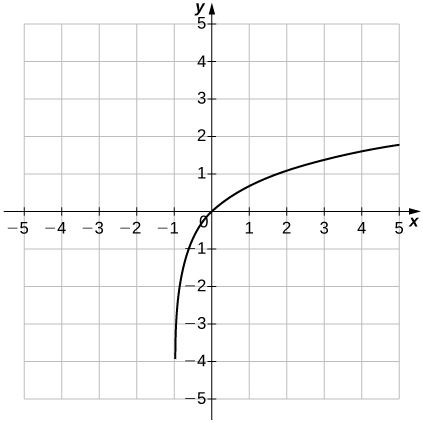#### Solution

Domain: $(-1,\infty)$, Range: $(-\infty ,\infty)$, Vertical asymptote at $x=-1$

For the following exercises, determine the domain of each function.

42. $f(x) =\frac{3e^x+5}{x+2}$

43. $f(x)=\frac{1}{e^x-2}$

#### Solution

Domain: $(-\infty,\ln2) \cup (\ln2, \infty)$

44. $g(x)=\frac{2x+1}{\sqrt{e^x-5}}$

45. $g(x)=\sqrt{\ln(x-2)-6}$

#### Solution

Domain: $[e^6+2, \infty)$

46. $f(x)=\frac{1}{\sqrt{\ln(x-5)}}$

47. $f(x)=\sqrt{\ln(x^2-1)}$

#### Solution

Domain: $(-\infty,-\sqrt{2}] \cup [\sqrt{2},\infty)$

For the following exercises, use properties of logarithms to write the expressions as a sum, difference, and/or product of logarithms.

48. $\log x^4y$

49. $\log_3 \frac{9a^3}{b}$

#### Solution

$2+3\log_3 a-\log_3 b$

50. $\ln a\sqrt{b}$

51. $\log_5 \sqrt{125xy^3}$

#### Solution

$\frac{3}{2}+\frac{1}{2}\log_5 x+\frac{3}{2}\log_5 y$

52. $\log_4 \frac{\sqrt{xy}}{64}$

53. $\ln(\frac{6}{\sqrt{e^3}})$

#### Solution

$-\frac{3}{2}+\ln 6$

For the following exercises, solve the exponential equation exactly.

54. $5^x=125$

55. $e^{3x}-15=0$

#### Solution

$\frac{\ln 15}{3}$

56. $8^x=4$

57. $4^{x+1}-32=0$

#### Solution

$\frac{3}{2}$

58. $3^{x/14}=\frac{1}{10}$

59. $10^x=7.21$

#### Solution

$\log 7.21$

60. $4\cdot 2^{3x}-20=0$

61. $7^{3x-2}=11$

#### Solution

$\frac{2}{3}+\frac{\log 11}{3\log 7}$

For the following exercises, solve the logarithmic equation exactly, if possible.

62. $\log_3 x=0$

63. $\log_5 x=-2$

#### Solution

$x=\frac{1}{25}$

64. $\log_4 (x+5)=0$

65. $\log(2x-7)=0$

#### Solution

$x=4$

66. $\ln\sqrt{x+3}=2$

67. $\log_6 (x+9)+\log_6 x=2$

#### Solution

$x=3$

68. $\log_4(x+2)-\log_4(x-1)=0$

69. $\ln x+\ln (x-2)=\ln 4$

#### Solution

$1+\sqrt{5}$

For the following exercises, use the change-of-base formula and either base 10 or base $e$ to evaluate the given expressions. Answer in exact form and in approximate form, rounding to four decimal places.

70. $\log_5 47$

71. $\log_7 82$

#### Solution

$\frac{\ln 82}{\ln 7} \approx 2.2646$

72. $\log_6 103$

73. $\log_{0.5} 211$

#### Solution

$\frac{\ln 211}{\ln 0.5} \approx -7.7211$

74. ${\text{log}}_{2}\pi$

75. $\log_{0.2} 0.452$

#### Solution

$\frac{\ln 0.452}{\ln 0.2} \approx 0.4934$

76. Rewrite the following expressions in terms of exponentials and simplify.

a. $2\cosh(\ln x)$

b. $\cosh 4x+\sinh 4x$

c. $\cosh 2x-\sinh 2x$

d. $\ln(\cosh x+\sinh x)+\ln(\cosh x-\sinh x)$

77. [T] The number of bacteria $N$ in a culture after $t$ days can be modeled by the function $N(t)=1300\cdot 2^{t/4}$. Find the number of bacteria present after 15 days.

#### Solution

$\approx 17,491$

78. [T] The demand $D$ (in millions of barrels) for oil in an oil-rich country is given by the function $D(p)=150\cdot 2.7^{-0.25p}$, where $p$ is the price (in dollars) of a barrel of oil. Find the amount of oil demanded (to the nearest million barrels) when the price is between $15 and$20.

79. [T] The accumulated amount $A$ of a $100,000 investment whose interest compounds continuously for $t$ years is given by $A(t)=100,000\cdot e^{0.055t}$. Find the amount $A$ accumulated in 5 years. #### Solution Approximately$131,653 is accumulated in 5 years.

80. [T] An investment is compounded monthly, quarterly, or yearly and is given by the function $A=P(1+\frac{j}{n})^{nt}$, where $A$ is the value of the investment at time $t, \, P$ is the initial principle that was invested, $j$ is the annual interest rate, and $n$ is the number of time the interest is compounded per year. Given a yearly interest rate of 3.5% and an initial principle of \$100,000, find the amount $A$ accumulated in 5 years for interest that is compounded a. daily, b., monthly, c. quarterly, and d. yearly.

81. [T] The concentration of hydrogen ions in a substance is denoted by $[\text{H}^{+}]$, measured in moles per liter. The pH of a substance is defined by the logarithmic function $\text{pH}=-\log[\text{H}^{+}]$. This function is used to measure the acidity of a substance. The pH of water is 7. A substance with a pH less than 7 is an acid, whereas one that has a pH of more than 7 is a base.

1. Find the pH of the following substances. Round answers to one digit.
2. Determine whether the substance is an acid or a base.
1. Eggs: $[\text{H}^{+}]=1.6 \times 10^{-8}$ mol/L
2. Beer: $[\text{H}^{+}]=3.16 \times 10^{-3}$ mol/L
3. Tomato Juice: $[\text{H}^{+}]=7.94 \times 10^{-5}$ mol/L

#### Solution

i. a. pH = 8 b. Base ii. a. pH = 3 b. Acid iii. a. pH = 4 b. Acid

82. [T] Iodine-131 is a radioactive substance that decays according to the function $Q(t)=Q_0\cdot e^{-0.08664t}$, where $Q_0$ is the initial quantity of a sample of the substance and $t$ is in days. Determine how long it takes (to the nearest day) for 95% of a quantity to decay.

83. [T] According to the World Bank, at the end of 2013 ($t=0$) the U.S. population was 316 million and was increasing according to the following model:

$P(t)=316e^{0.0074t}$,

where $P$ is measured in millions of people and $t$ is measured in years after 2013.

1. Based on this model, what will be the population of the United States in 2020?
2. Determine when the U.S. population will be twice what it is in 2013.

#### Solution

a. $\approx 333$ million b. 94 years from 2013, or in 2107

84. [T] The amount $A$ accumulated after 1000 dollars is invested for $t$ years at an interest rate of 4% is modeled by the function $A(t)=1000(1.04)^t$.

1. Find the amount accumulated after 5 years and 10 years.
2. Determine how long it takes for the original investment to triple.

85. [T] A bacterial colony grown in a lab is known to double in number in 12 hours. Suppose, initially, there are 1000 bacteria present.

1. Use the exponential function $Q=Q_0e^{kt}$ to determine the value $k$, which is the growth rate of the bacteria. Round to four decimal places.
2. Determine approximately how long it takes for 200,000 bacteria to grow.

#### Solution

a. $k \approx 0.0578$ b. $\approx 92$ hours

86. [T] The rabbit population on a game reserve doubles every 6 months. Suppose there were 120 rabbits initially.

1. Use the exponential function $P=P_0 a^t$ to determine the growth rate constant $a$. Round to four decimal places.
2. Use the function in part a. to determine approximately how long it takes for the rabbit population to reach 3500.

87. [T] The 1906 earthquake in San Francisco had a magnitude of 8.3 on the Richter scale. At the same time, in Japan, an earthquake with magnitude 4.9 caused only minor damage. Approximately how much more energy was released by the San Francisco earthquake than by the Japanese earthquake?

#### Solution

The San Francisco earthquake had $10^{3.4}$ or $\approx 2512$ times more energy than the Japan earthquake.

## Glossary

base
the number $b$ in the exponential function $f(x)=b^x$ and the logarithmic function $f(x)=\log_b x$
exponent
the value $x$ in the expression $b^x$
hyperbolic functions
the functions denoted $\sinh, \, \cosh, \, \tanh, \, \text{csch}, \, \text{sech}$, and $\coth$, which involve certain combinations of $e^x$ and $e^{-x}$
inverse hyperbolic functions
the inverses of the hyperbolic functions where $\cosh$ and $\text{sech}$ are restricted to the domain $[0,\infty)$; each of these functions can be expressed in terms of a composition of the natural logarithm function and an algebraic function
natural exponential function
the function $f(x)=e^x$
natural logarithm
the function $\ln x=\log_e x$
number e
as $m$ gets larger, the quantity $(1+(1/m))^m$ gets closer to some real number; we define that real number to be $e$; the value of $e$ is approximately 2.718282